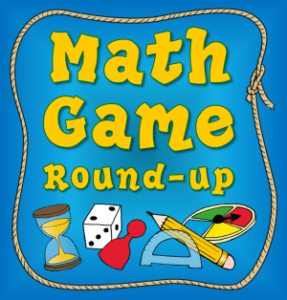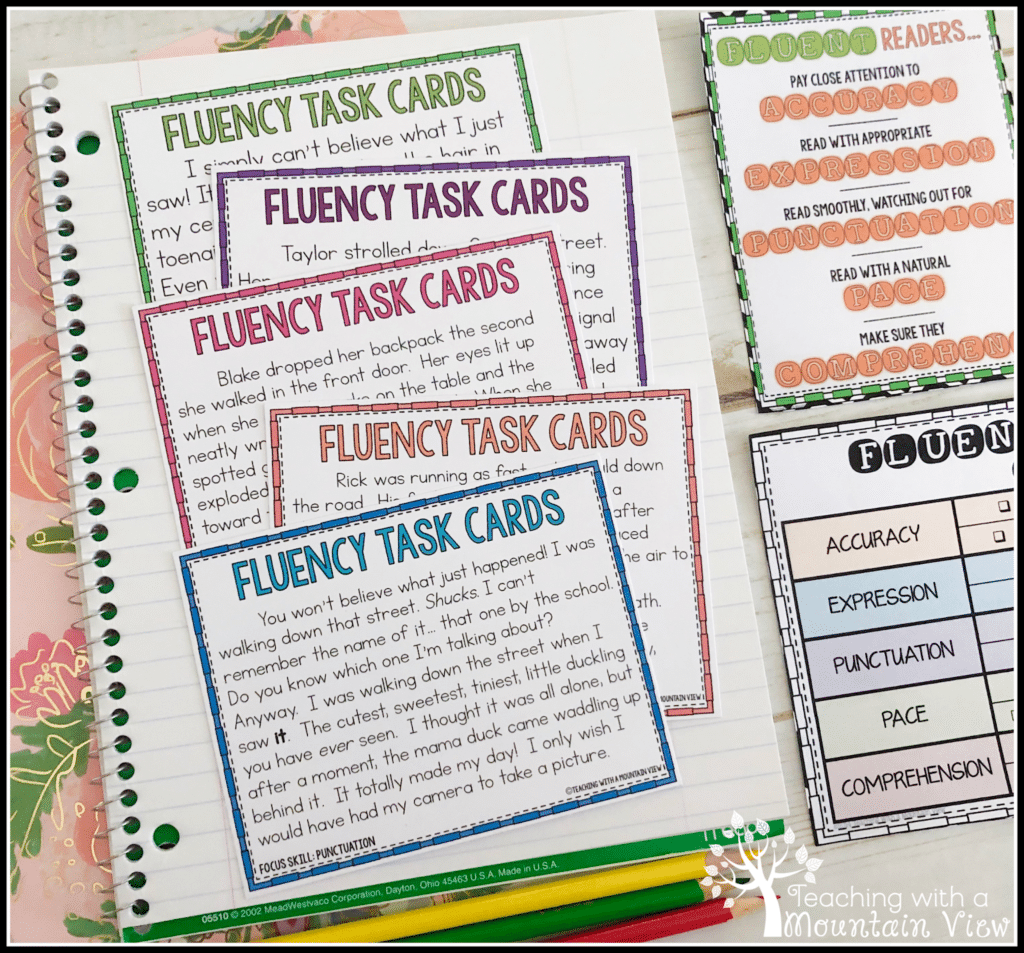# Dividing Fractions Anchor Chart, Game Freebie, and Math Journal

We tackled dividing fractions this week!  I was a little bit apprehensive about it since these are wee little fourth graders working on this tough stuff, but they did GREAT!  Although I follow the CCSS, I thought it was a little silly that they don't include dividing a fraction by a fraction at fifth grade since it's easy enough to add in once they know how to divide a whole number by a fraction and a fraction by a whole number, so we just did it all.  And we tossed in mixed numbers while they were at it. Rigor!We started with a math journal entry about dividing fractions with visual models.  Funny story– I spent HOURS making a pretty study guide for this, and my sweet husband just didn't understand it.  He (like most of us) is old-fashioned, and we don't feel the need to understand the “why” behind most of these math concepts.  But my kids rocked this!  It clicked for them right away.  Thank goodness!  You can see the math journal page I created with them in this picture.  I don't really emphasize the visual models, but I think it helps them to see why dividing a whole number by a fraction gives them a larger number and vice-versa.

Then we got down to the nitty gritty.  We introduced reciprocals first using our Funny & Fabulous Fraction Stories book, and then we did a step-by-step dividing fractions anchor chart.  You can see that we included the directions for  dividing fractions by fractions, dividing whole numbers by fractions, and dividing fractions by whole numbers.At the bottom, we noted the “Keep-Change-Flip” shortcut.  They copied this in their math journal as well.  We have already referenced it several times. (For instance, a student comes and asks me why they got an answer wrong, and it's easy to say, “what step did you forget?”  Problem solves 99% of the time.)The second day, we worked on some dividing fractions games.  I'm linking up with the fabulous Laura Candler and her Math Game Round-Up.  Check it out for more FREE math game ideas from numerous teacher bloggers!There are two versions of the dividing fractions game–I had half of my group working on one while they other half worked on the other.  One uses pattern blocks, and the other uses dice-in-dice, but could easily be adapted to use with several regular dice.

Breaking Up the Blocks was great because it helped students review fractional parts and making fractions.  They choose 2 blocks, then use the fractional parts (i.e. a triangle is 1/6 of a whole hexagon) to form equations.  Then, they choose 5 blocks, creating a mixed number (or whole number) and a fraction and divide them.  The kids LOVED it.  Definitely better than an ordinary worksheet practicing problems!Dividing the Dice uses dice in dice, always a favorite among kids.  They roll two of them and then use the dice to form fractions, and then we added in a regular dice to make mixed numbers (or whole numbers if you aren't working with mixed numbers).  Like I said, if you don't have dice in dice, you can just have kids roll two dice twice.  These are simple fraction games, but they were incredibly engaging and meaningful to the kids.(If you notice, the students in the pictures have worksheets that are slightly different that the one I'm posting.  Proof that my goodies are student-tested!  After they used them, I made a few changes to make it more clear before posting them for you all to use.)

Finally, we are working on our dividing fractions task cards.  There are three sets to practice all the different types of dividing fractions.  Fun, fun!We are so close to winding up our units and starting on our end of the year projects.  I CAN'T WAIT!

Are you teaching more than just dividing fractions, or do your students need more activities for operations with fractions?  Stop by my store to see the Ultimate Fraction Operations Resource Bundle!## Enter your email to download everything you need to implement five days of fluency lessons-- completely FREE!